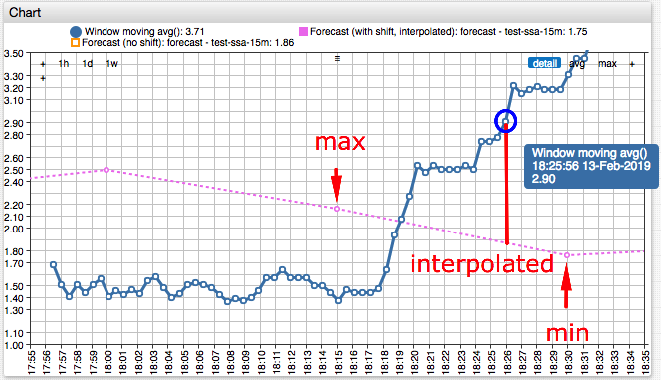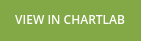# # Statistical Forecast Functions

## # Reference

### #`forecast()`

``````forecast([string name]) object
``````

Returns forecast object for the entity, metric, and tags in the current window. The object contains neighboring forecast periods based on `time` field calculated by subtracting 50% of the forecast period from the window center (`windowTime`).

If the `name` is specified, the forecast object is retrieved for the named forecast settings.

The object contains the following fields:

• `previous` - Previous period value.
• `next` - Next period value.
• `min` - Minimum of the previous and the next period values.
• `max` - Maximum of the previous and the next period values.
• `linear` - Linearly interpolated value between previous and next periods based on `time` field.
• `windowTime` - Window center time calculated as average of first and last timestamps in the current window.
• `time` - Forecast time calculated as `windowTime - 0.50*forecast_series_period`.
• `previousTime` - Previous period start time.
• `nextTime` - Next period start time.
Parameter Time Date
`command.time` `1550082342000` `2019-02-13 18:25:56`
`previousTime` `1550080800000` `2019-02-13 18:00:00`
`nextTime` `1550081700000` `2019-02-13 18:15:00`
`windowTime` `1550081922000` `2019-02-13 18:18:42`
`time` `1550081472000` `2019-02-13 18:11:12`

The `violates()` function in the forecast object returns `true` if the input value deviates by more than the specified delta from the `min` and `max` range. The delta must be a non-negative number.

``````violates(double a, double delta) {
return a < min - delta || a > max + delta;
}
``````Example:

``````violates(avg(), 10)
``````

### #`forecast_stdev`

``````forecast_stdev() double
``````

Returns forecast standard deviation.

### #`forecast_score_stdev`

Returns the standard deviation of actual aggregated values from the best fitting forecast values within the scoring interval.

Scoring is a procedure that identifies parameters that produce the best fitting forecast with the smallest standard deviation from observed aggregated values.

``````forecast_score_stdev(string name) double
``````

### #`forecast_score_deviation`

``````forecast_score_deviation(string name, double a) double
``````

Returns the difference between a number `a` (such as the last value, or moving average `avg()`) and the `forecast().interpolated` value, divided by the forecast score standard deviation.

The function returns `NaN` if either forecast or forecast score standard deviation cannot be retrieved, or if the standard deviation is zero.

The formula is:

``````(a - forecast(name).interpolated/forecast_score_stdev(name)
``````

### #`forecast_deviation`

``````forecast_deviation(number a) double
``````

Returns difference between a number `a` and the `forecast().interpolated` value, divided by the forecast standard deviation.

``````(a - forecast().interpolated)/forecast_stdev()
``````

### #`thresholdTime`

``````thresholdTime(number min, number max [, string interval]) long
``````

Returns Unix time in milliseconds when the stored forecast value is outside of the `(min, max)` range for the first time during time interval `interval`.

`interval` is specified as count and unit, for example `1 WEEK`. If `interval` is not specified, the function checks all forecast values in the future.

Thresholds `min` and `max` are ignored by the function if either is set to `null`. If both `min` and `max` are specified, the following rules apply:

• If threshold `max` is equal or greater than `min`, the function returns time when the forecast value is outside of the specified range.
• If threshold `min` exceeds `max`, the function returns time when the forecast value is within the specified range.

The function returns `null` if no stored forecast is found in the database.

### # Example

``````/* Returns time, within the next 6 hours, when the forecast exceeds 90.
Returns `null`, if all forecast values are below 90 or the violation occurs after the `6 HOUR` window. */
thresholdTime(null, 90, '6 HOUR')
``````

### # Example Table

Current time is `2018-Jul-07 15:00`, or `1530975600000` in Unix time (milliseconds).

Forecast Values:

Value Date Time Note
`70` `2018-Jul-08 15:00` `1530975600000` 1 day from `now`
`80` `2018-Jul-09 15:00` `1531148400000` 2 days from `now`
`90` `2018-Jul-10 15:00` `1531234800000` 3 days from `now`
`100` `2018-Jul-16 15:00` `1531753200000` 9 days from `now`
``````/* Returns 1531753200000 (2018-Jul-16 15:00)
First time when the forecast 100 exceeds 'max' threshold 95 */
thresholdTime(null, 95)
``````
``````/* Returns null. No forecast value exceeds 120. */
thresholdTime(null, 120)
``````
``````/* Returns null. No forecast value exceeds 95 within the next 7 days, by 2018-Jul-14 15:00. */
thresholdTime(null, 95, '7 DAY')
``````
``````/* Returns 1531234800000 (2018-Jul-10 15:00).
First time when the forecast 90 exceeds 'max' threshold 80.
Forecast 80 on 2018-Jul-09 15:00 is ignored as not exceeding the threshold. */
thresholdTime(null, 80, '7 DAY')
``````
``````/* Returns 1530975600000 (2018-Jul-08 15:00).
First time when the forecast 70 is below 'min' threshold 80. */
thresholdTime(80, null, '7 DAY')
``````
``````/* Returns 1531753200000 (2018-Jul-16 15:00)
Outside range check: case when 'max' threshold is greater than 'min' threshold.
First time when the forecast 100 is either below 70 OR above 90. */
thresholdTime(70, 90)
``````
``````/* Returns 1531148400000 (2018-Jul-09 15:00)
Within range check: case when 'min' threshold is greater than 'max' threshold.
First time when the forecast 80 is BOTH above 'max' threshold 70 AND below 'min' threshold 90. */
thresholdTime(90, 70)
``````
``````/* Returns 1531148400000 (2018-Jul-09 15:00)
Case when 'min' threshold equals 'max' threshold.
First time when the forecast 80 is either below 70 (false) OR above 70 (true). */
thresholdTime(70, 70)
``````